Question

# A sample of final exam scores is normally distributed with a mean equal to 20 and...

A sample of final exam scores is normally distributed with a mean equal to 20 and a variance equal to 16.

• Part (a)

What percentage of scores are between 16 and 24? (Round your answer to two decimal places.)
%

• Part (b)

What raw score is the cutoff for the top 10% of scores? (Round your answer to one decimal place.)

• Part (c)

What is the proportion below 15? (Round your answer to four decimal places.)

• Part (d)

What is the probability of a score less than 25? (Round your answer to four decimal places.)#### Earn Coins

Coins can be redeemed for fabulous gifts.

Similar Homework Help Questions
• ### A sample of final exam scores is normally distributed with a mean equal to 29 and...

A sample of final exam scores is normally distributed with a mean equal to 29 and a variance equal to 16. Part (a) What percentage of scores are between 25 and 33? (Round your answer to two decimal places.) Part (b) What raw score is the cutoff for the top 10% of scores? (Round your answer to one decimal place.) Part (c) What is the proportion below 23? (Round your answer to four decimal places.) Part (d) What is the...

• ### A sample of final exam scores is normally distributed with a mean equal to 22 and a variance equal to 25. Part (a) Wha...

A sample of final exam scores is normally distributed with a mean equal to 22 and a variance equal to 25. Part (a) What percentage of scores are between 17 and 27? (Round your answer to two decimal places.) % Part (b) What raw score is the cutoff for the top 10% of scores? (Round your answer to one decimal place.) Part (c) What is the proportion below 14? (Round your answer to four decimal places.) Part (d) What is...

• ### Exam grades: Scores on a statistics final in a large class were normally distributed with a...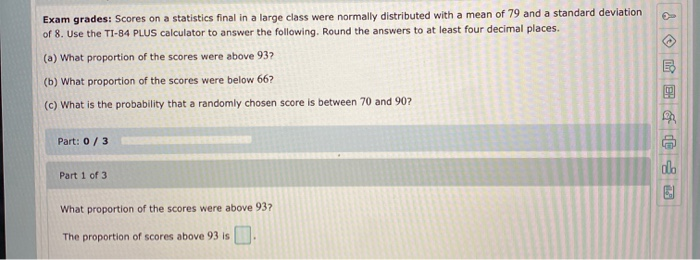Exam grades: Scores on a statistics final in a large class were normally distributed with a mean of 79 and a standard deviation of 8. Use the TI-84 PLUS calculator to answer the following. Round the answers to at least four decimal places. (a) What proportion of the scores were above 932 (b) What proportion of the scores were below 66? (c) What is the probability that a randomly chosen score is between 70 and 90? Part: 0/3 Part 1...

• ### Exam grades: Scores on a statistics final in a large class were normally distributed with a...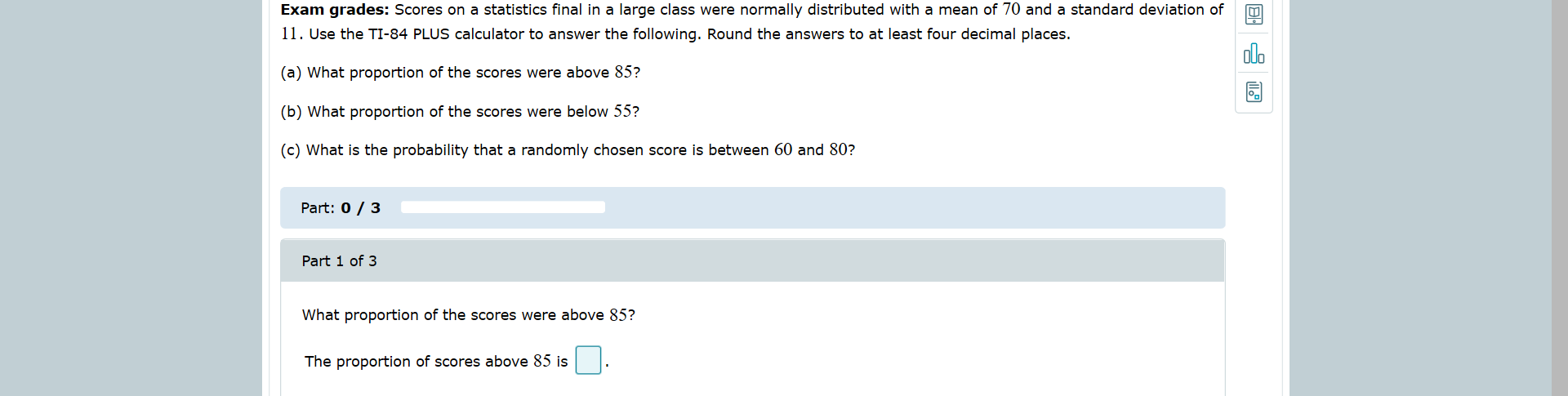Exam grades: Scores on a statistics final in a large class were normally distributed with a mean of 70 and a standard deviation of 11. Use the TI-84 PLUS calculator to answer the following. Round the answers to at least four decimal places. (a) What proportion of the scores were above 85? (b) What proportion of the scores were below 55? (c) What is the probability that a randomly chosen score is between 60 and 80? Part: 0/3 Part 1...

• ### The scores on a psychology exam were normally distributed with a mean of 62 and a...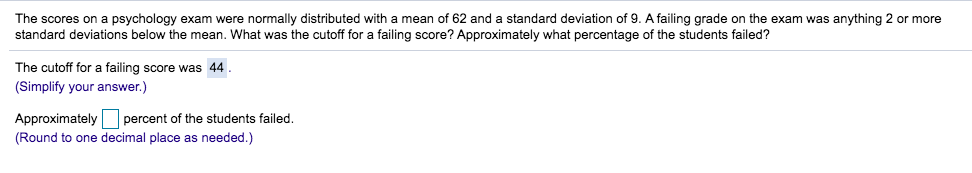The scores on a psychology exam were normally distributed with a mean of 62 and a standard deviation of 9. A failing grade on the exam was anything 2 or more standard deviations below the mean. What was the cutoff for a failing score? Approximately what percentage of the students failed? The cutoff for a failing score was 44. (Simplify your answer.) Approximately percent of the students failed. (Round to one decimal place as needed.)

• ### Exam grades: Scores on a statistics final in a large class were normally distributed with a mean of 79 and a standa...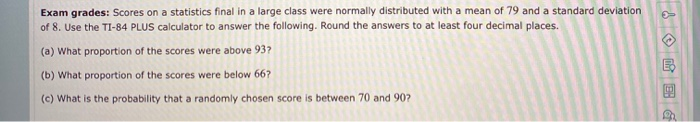Exam grades: Scores on a statistics final in a large class were normally distributed with a mean of 79 and a standard deviation of 8. Use the TI-84 PLUS calculator to answer the following. Round the answers to at least four decimal places. (a) What proportion of the scores were above 93? (b) What proportion of the scores were below 66? (c) What is the probability that a randomly chosen score is between 70 and 90?

• ### The scores on a psychology exam were normally distributed with mean of 55 and a standard...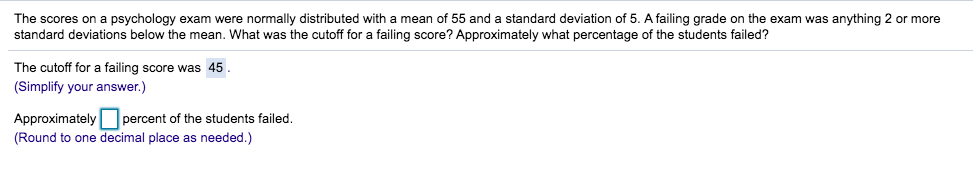The scores on a psychology exam were normally distributed with mean of 55 and a standard deviation of 5. A failing grade on the exam was anything 2 or more standard deviations below the mean. What was the cutoff for a failing score? Approximately what percentage of the students failed? The cutoff for a failing score was 45. (Simplify your answer.) Approximately percent of the students failed. (Round to one decimal place as needed.)

• ### ​The scores on a psychology exam were normally distributed with a mean of 58 and a...

​The scores on a psychology exam were normally distributed with a mean of 58 and a standard deviation of 6. A failing grade on the exam was anything 2 or more standard deviation below the mean. What was the cutoff for a failing score? Approximately what percentage of the students failed? The cutoff for a failing score was ____ Simplify answer) Approximately ___ percent of the students failed ( Round to one decimal place as needed)

• ### Problem 3: Scores on an exam are assumed to be normally distributed with mean /u =...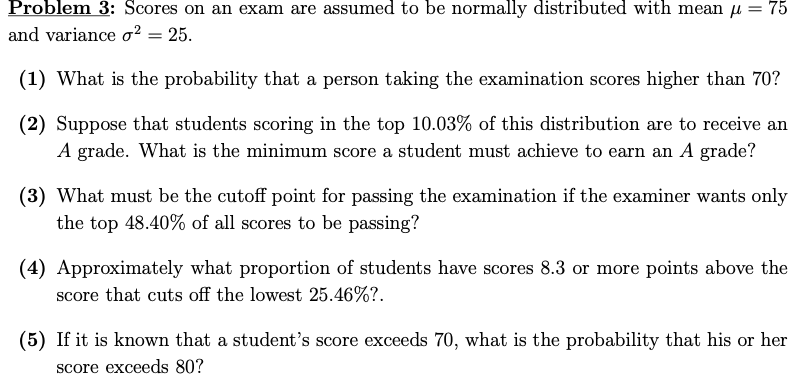Problem 3: Scores on an exam are assumed to be normally distributed with mean /u = 75 and variance a2 = 25 (1) What is the probability that a person taking the examination scores higher than 70? (2) Suppose that students scoring in the top 10.03% of this distribution are to receive an A grade. What is the minimum score a student must achieve to earn an A grade? (3) What must be the cutoff point for passing the examination...

• ### Scores on a chemistry final exam are normally distributed with a mean of 280 and a...

Scores on a chemistry final exam are normally distributed with a mean of 280 and a standard deviation of 20. Determine the percentage of samples of size 4 that will have mean scores within 14 points of the population mean score of 280. Round to two decimal places. A. 99.48 % B. 83.84 % C. 91.92 % D. 51.60 %